Angles: an angle is the figure formed by two rays, called the sides of the angle, sharing a common endpoint, called the vertex of the angle.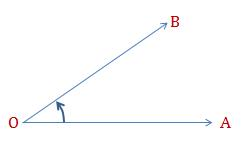Two rays$\overrightarrow{OA}$ and$\overrightarrow{OB}$  and  having a common point will form an angle AOB which is written as$\angle AOB$.$OA$ and$OB$ are called the arms of the angle and O is called the vertex of the angle$\angle AOB$.

Measure of an Angle: It is the amount of rotation through which one arm of the angle has to be rotated, about the vertex, to bring it to the position of the other arm.

Angle is measured in degrees, denoted by${\ }^{\circ}$.

A complete rotation around a point makes an angle of$360^{\circ}$.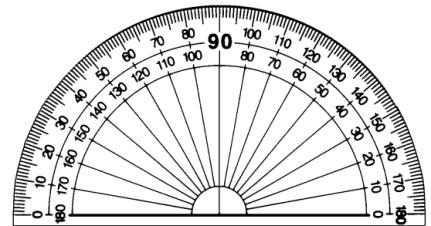One degree$1^{\circ}=60$ minutes (also written as$60^{'}$ ).

One Minute$1^{'}=60$ seconds (also written as$60^{''}$ ).

To draw angles, the commonly used equipment is called protector.

Kinds of Angles

 Name of the Angle Description Diagram Acute Angle An angle whose measure is more than$0^{\circ}$$0^{\circ}$ but less than$90^{\circ}$$90^{\circ}$ is called an acute angle.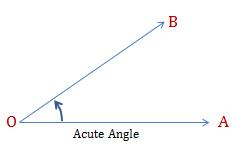Right Angle An angle whose measure is equal to$90^{\circ}$$90^{\circ}$ is called a right angle.Obtuse Angle An angle whose measure is more than$90^{\circ}$$90^{\circ}$ but less than$180^{\circ}$$180^{\circ}$ is called an obtuse angle.Straight Angle An angle whose measure is equal to$180^{\circ}$$180^{\circ}$ is called a straight angle.Reflex Angle An angle whose measure is more than$180^{\circ}$$180^{\circ}$ but less than$360^{\circ}$$360^{\circ}$ is called a reflex angle.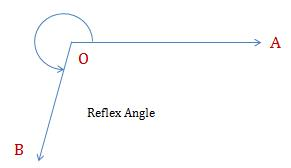Complete Angle An angle whose measure is equal to$360^{\circ}$$360^{\circ}$ is called a complete angle.Equal Angles: Two angles are said to be equal if they have the same measure.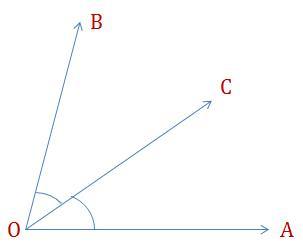Bisector of an Angle: Any ray is called a bisector of an angle if$\angle AOC=\angle COB$ .

Complimentary Angles: If the sum of two angles is$90^{\circ}$, then the angles are called complimentary angles. We can also say that Complementary angles are angle pairs whose measures sum to one right angle.

Supplementary Angles:  If the sum of two angles is$180^{\circ}$, then the angles are called supplementary angles. If the two supplementary angles are adjacent their non-shared sides form a straight line.

Adjacent Angles: If two angles share one common arm and a common vertex in such a way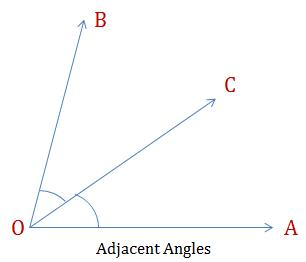that the other angle arms are on either side of the common arm then they are called adjacent angles.  In this example we see that$O$ is the common vertex, and$OC$ is the common arm. Hence we can say that$\angle AOC \ \&\ \angle COB$ are adjacent angles.

Linear Pair of Angles: If the adjacent angles are such that the, the non-common arms form a straight angle, then the angles are called linear pair of angles.  In this case$\angle AOC+\angle COB=180^{\circ}=\angle AOC$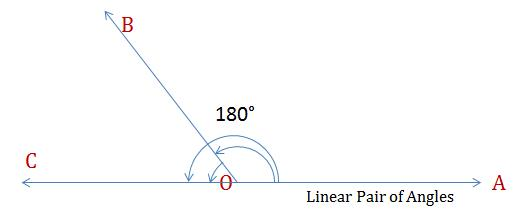Another way of looking at this is that is the sum of two adjacent angles is$180^{\circ}$, then they will form a linear pair of angles.

One more important result that you should know is that the sum of angles around a point (or dot) is$360^{\circ}$.

Vertically Opposite Angles: When two straight lines intersect at one point, they will form vertically opposite angles which are equal.

As you see, lines$AB\ and\ CD$ intersect at point$O$ . It forms two pairs of vertically opposite angles, which are:$\angle AOD \ \& \ \angle COB$ are vertically opposite$\angle AOC \ \&\ \angle DOB$ are vertically opposite

We can also prove that these angles are equal to each other.

Given: Line$AB \ and\ CD$  intersect at point$O$

To Prove: i)$\angle BOC=\angle AOD$ and ii)$\angle AOC=\angle DOB$

Proof: Since ray$OC$ stands on a straight line$\angle AOC+\angle BOC = 180^{\circ}$          [Linear Pair Axiom]

Similarly, since ray$OA$ stands on line$CD$$\angle AOC+\angle AOD=180^{\circ}$         [Linear Pair Axiom]

Therefore$\angle AOC+\angle BOC=\angle AOC+\angle AOD$

Or$\angle BOC=\angle AOD$. Hence proved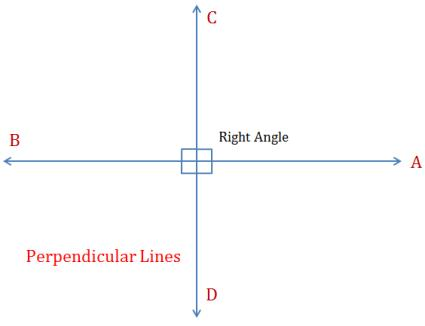Similarly, you can prove$\angle AOC=\angle DOB$

Perpendicular Lines: A line is said to be perpendicular to another line if the two lines intersect at a right angle. If$AB \ and\ CD$ are two perpendicular lines, then they are denoted as$AB \perp CD$.